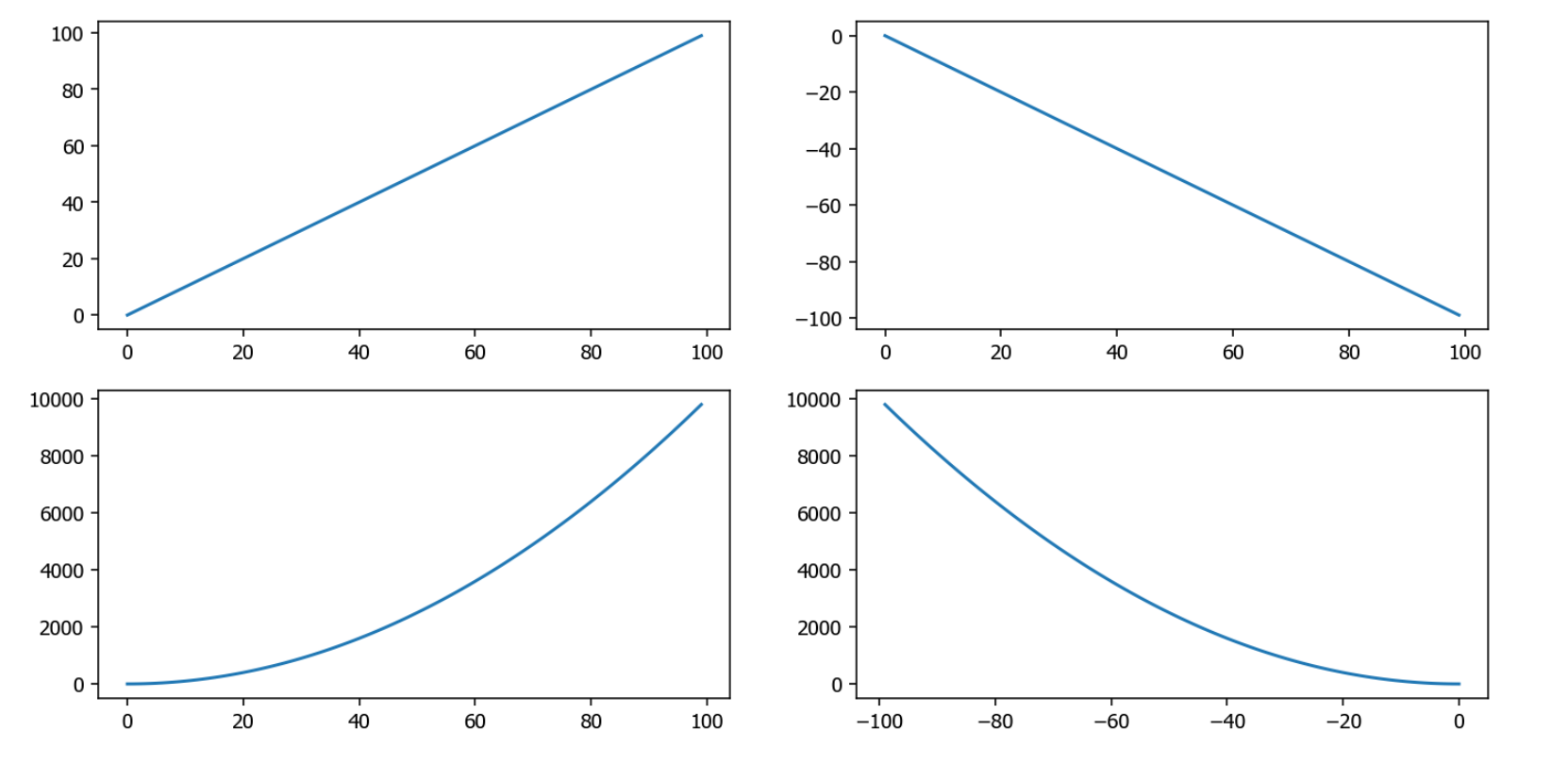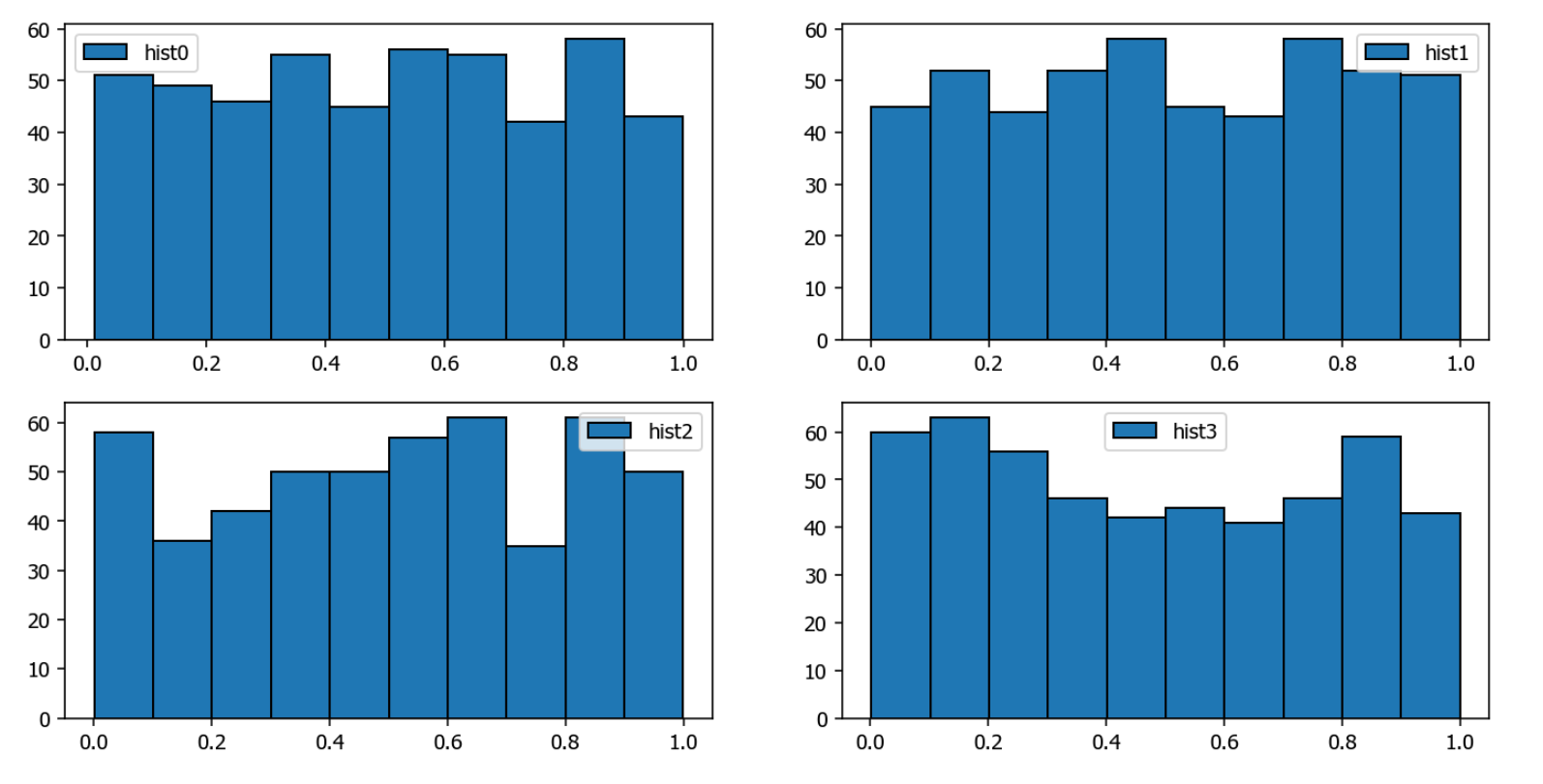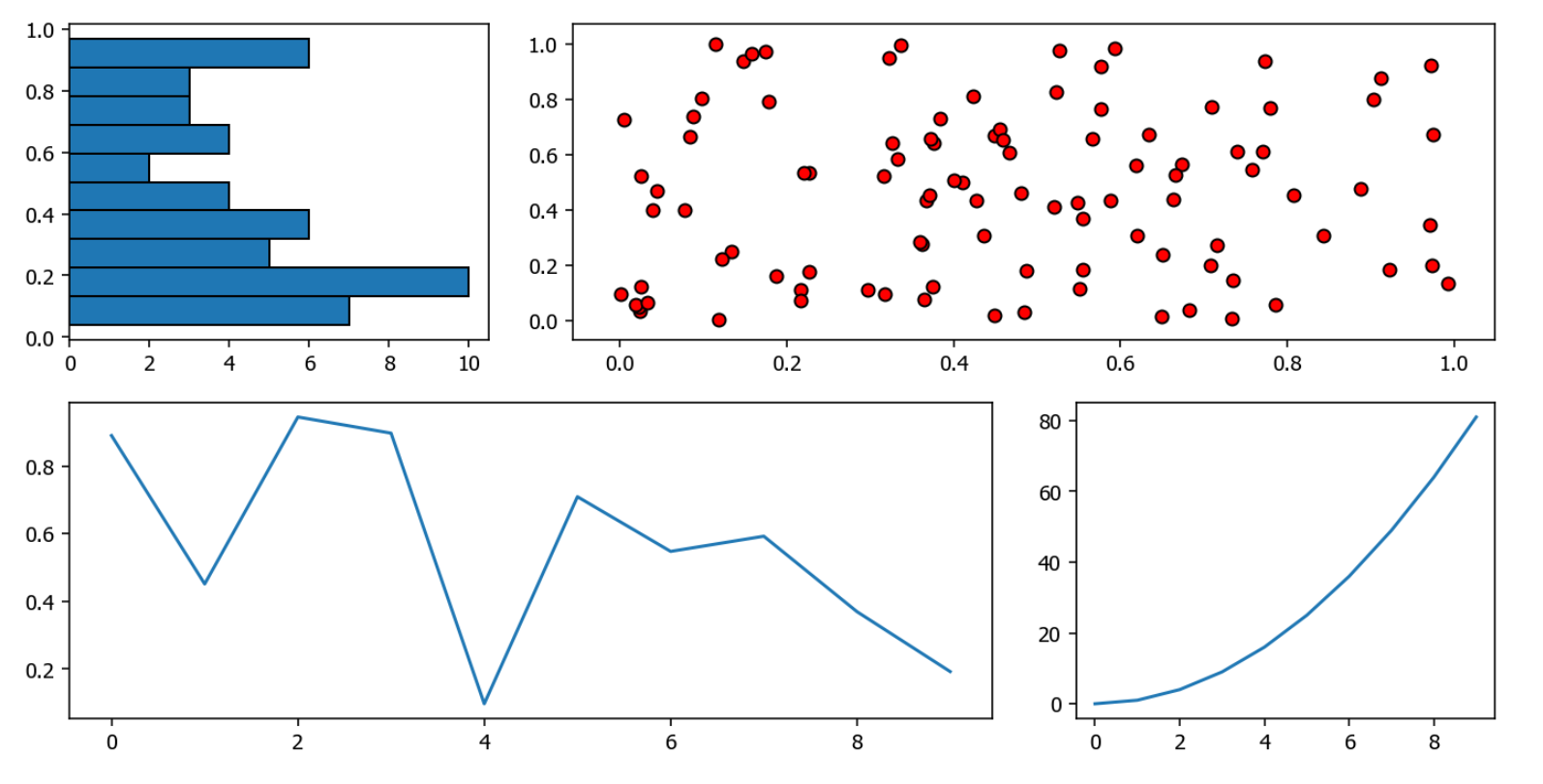# Anders Wang

import numpy as np
import matplotlib.pyplot as plt

x = np.arange(100)

# 创建图像布局对象fig
fig = plt.figure(figsize = (12, 6))

# 221代表创建2行2列一共4个子图，并从左往右第1个子图开始绘图。
ax1.plot(x, x)

ax2.plot(x, -x)

ax3.plot(x, x ** 2)

ax4.plot(-x, x ** 2)

plt.show()• 使用pyplot方式直接创建子图

import numpy as np
import matplotlib.pyplot as plt

x = np.arange(100)

plt.figure(figsize = (12, 6))
# 先画好子图具体位置
plt.subplot(221)
# 在该子图下绘图
plt.plot(x, x)

plt.subplot(222)
plt.plot(x, -x)

plt.subplot(223)
plt.plot(x, x ** 2)

plt.subplot(224)
plt.plot(-x, x ** 2)

plt.show()

• 使用subplots创建多个子图

import numpy as np
import matplotlib.pyplot as plt

# 将画布分割为2行2列，起始值为0。
fig, axes = plt.subplots(figsize = (12, 6), nrows = 2, ncols = 2)
# 给第1行第1列绘图
axes.hist(np.random.rand(500), label = 'hist0', edgecolor = 'black')
# 给图形添加标签
axes.legend()

axes.hist(np.random.rand(500), label = 'hist1', edgecolor = 'black')
axes.legend()

axes.hist(np.random.rand(500), label = 'hist2', edgecolor = 'black')
axes.legend()

axes.hist(np.random.rand(500), label = 'hist3', edgecolor = 'black')
axes.legend()

plt.show()• plt.GridSpec：实现更复杂的排列方式
import numpy as np
import matplotlib.pyplot as plt

# 声明一个GridSpec对象实例，创建的是2行3列的图像布局。
grid = plt.GridSpec(nrows=2, ncols=3, wspace=0.2, hspace=0.2)

# 设置整个图像大小。
plt.figure(figsize = (12, 6))
# 第一个子图的具体排列位置为(0,0)。
plt.subplot(grid[0, 0])
plt.hist(np.random.rand(50), orientation='horizontal', edgecolor = 'black')

plt.subplot(grid[0, 1:])
plt.scatter(np.random.rand(100),np.random.rand(100), color = 'r', edgecolor = 'black')

plt.subplot(grid[1, :2])
plt.plot(np.arange(10), np.random.rand(10))

plt.subplot(grid[1, 2]);
plt.plot(np.arange(10), np.arange(10) ** 2)

plt.show()[1, 2, 3]
[4, 5, 6]